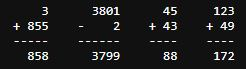# Help Arithmetic Arranger

Hi I tested my code on the Arithmetic Arranger project and it seems fine but it does not pass the test, can you help me find what’s wrong?

``````problems=["32 + 698", "3801 - 2", "45 + 43", "123 + 49"]

def arithmetic_arranger(problems, flag=False):
line1 = line2 = line3 = line4 = ""

if len(problems)>5:
return print("Error: Too many problems.")

for problem in problems:
words = problem.split()

num1 = words
op = words
num2 = words
bar_len = len(max(num1,num2))+2

if len(op)!=1 or op not in ['+','-']:
return print("Error: Operator must be '+' or '-'.")

if not (num1.isdigit() and num2.isdigit()):
return print("Error: Numbers must only contain digits.")

if not (len(num1)<=4 and len(num2)<=4):
return print("Error: Numbers cannot be more than four digits.")

if op == '+':
ris = int(num1)+int(num2)
elif op == '-':
ris = int(num1)-int(num2)

line1 += " "*(bar_len-len(str(num1))) + str(num1) + " "*4
line2 += op + " "*(bar_len-len(str(num2))-1) + str(num2) + " "*4
line3 += "-"*(bar_len)                            + " "*4
line4 += " "*(bar_len-len(str(ris))) + str(ris)  + " "*4

if flag == False:
return print(line1[:-4] + "\n" + line2[:-4] + "\n" + line3[:-4])
else:
return print(line1[:-4] + "\n" + line2[:-4] + "\n" + line3[:-4] + "\n" + line4[:-4])

arithmetic_arranger(problems,True)
``````

here’s the log:

`````` python main.py
32      3801      45     123
+ 698    -    2    + 43    + 49
-----    ------    ----    ----
F..F..
======================================================================
FAIL: test_arrangement (test_module.UnitTests)
----------------------------------------------------------------------
Traceback (most recent call last):
File "/home/runner/boilerplate-arithmetic-formatter/test_module.py", line 10, in test_arrangement
self.assertEqual(actual, expected, 'Expected different output when calling "arithmetic_arranger()" with ["3 + 855", "3801 - 2", "45 + 43", "123 + 49"]')
AssertionError: '    [19 chars]     123\n+ 855    -    2    + 43    + 49\n---[24 chars]----' != '    [19 chars]      123\n+ 855    -    2    + 43    +  49\n-[27 chars]----'
-     3      3801      45     123
+     3      3801      45      123
?                        +
- + 855    -    2    + 43    + 49
+ + 855    -    2    + 43    +  49
?                             +
- -----    ------    ----    ----+ -----    ------    ----    -----?                                +
: Expected different output when calling "arithmetic_arranger()" with ["3 + 855", "3801 - 2", "45 + 43", "123 + 49"]

======================================================================
FAIL: test_solutions (test_module.UnitTests)
----------------------------------------------------------------------
Traceback (most recent call last):
File "/home/runner/boilerplate-arithmetic-formatter/test_module.py", line 39, in test_solutions
self.assertEqual(actual, expected, 'Expected solutions to be correctly displayed in output when calling "arithmetic_arranger()" with arithmetic problems and a second argument of `True`.')
AssertionError: '   3[19 chars]     123\n- 698    - 3801    + 43    + 49\n---[57 chars] 172' != '   3[19 chars]      123\n- 698    - 3801    + 43    +  49\n-[61 chars] 172'
-    32         1      45     123
+    32         1      45      123
?                        +
- - 698    - 3801    + 43    + 49
+ - 698    - 3801    + 43    +  49
?                             +
- -----    ------    ----    ----
+ -----    ------    ----    -----
?                            +
-  -666     -3800      88     172+  -666     -3800      88      172?                        +
: Expected solutions to be correctly displayed in output when calling "arithmetic_arranger()" with arithmetic problems and a second argument of `True`.

----------------------------------------------------------------------
Ran 6 tests in 0.005s

FAILED (failures=2)
``````

It looks like you have some trouble in spacing

I thought so, but I don’t get why, this is the output on my machine:found it!
I failed to measure the length of the dash line

` bar_len = len(max(num1,num2))+2`
corrected with
` bar_len = max(len(num1),len(num2))+2`

thank you anyway

This topic was automatically closed 182 days after the last reply. New replies are no longer allowed.